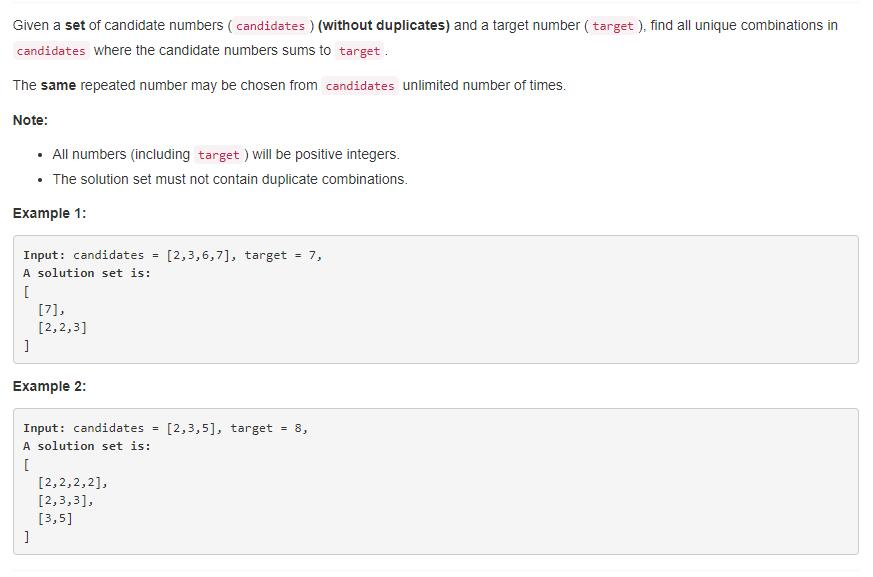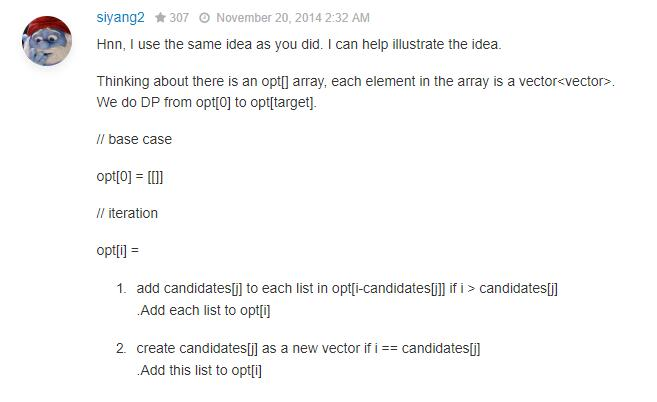# 题目描述（中等难度）# 解法一 回溯法

public List<List<Integer>> combinationSum(int[] nums, int target) {
List<List<Integer>> list = new ArrayList<>();
backtrack(list, new ArrayList<>(), nums, target, 0);
return list;
}

private void backtrack(List<List<Integer>> list, List<Integer> tempList, int [] nums, int remain, int start){
if(remain < 0) return;
else if(remain == 0) list.add(new ArrayList<>(tempList));
else{
for(int i = start; i < nums.length; i++){
backtrack(list, tempList, nums, remain - nums[i], i);
//找到了一个解或者 remain < 0 了，将当前数字移除，然后继续尝试
tempList.remove(tempList.size() - 1);
}
}
}


# 解法二 动态规划opt，表示和为 0 的所有情况的组合。

opt，表示和为 1 的所有情况的组合。

opt，表示和为 2 的所有情况的组合。

...

opt[target]，表示和为 target 的所有情况的组合，也就是题目所要求的。

• 如果 sum 刚好等于 nums [ i ]，那么就直接把 nums [ i ] 加到 list 里，算作一种情况。

例如 nums = [ 2, 3, 6, 7 ] , target = 7。

当求 sum = 3 的时候，也就是求 opt [ 3 ] 的时候，此时当遍历到 nums [ 1 ]，此时 nums [ 1 ] == sum == 3，所以此时 opt [ 3 ] = [ [ 3 ] ]。

• 如果 sum 大于 nums [ i ]，那么我们就把 opt [ sum - nums [ i ] ] 的所有情况都加上 nums [ i ] 然后作为 opt [ sum ] 。

例如 nums = [ 1, 2, 3, 6, 7 ] , target = 7。

当 sum 等于 5 的时候，也就是求 opt [ 5 ] 的时候，此时当遍历到 nums [ 1 ]，此时 nums [ 1 ] = 2 < sum。然后，就看 opt [ sum - nums [ i ] ] = opt [ 5 - 2 ] = opt [ 3 ]，而 opt [ 3 ] 在之前已经求好了，opt [ 3 ] = [ [ 1, 2 ], [ 3 ] ]，然后把 opt [ 3 ] 中的每一种情况都加上 nums [ 1 ] ，也就是 2，就变成了 [ [ 1, 2, 2 ], [ 3, 2 ] ]，这个就是遍历到 nums [ 1 ] 时候的 opt [ 5 ]了。

private List<List<Integer>> removeDuplicate(List<List<Integer>> list) {
Map<String, String> ans = new HashMap<String, String>();
for (int i = 0; i < list.size(); i++) {
List<Integer> l = list.get(i);
Collections.sort(l);
String key = "";
//[ 2 3 4] 转为 "2,3,4"
for (int j = 0; j < l.size() - 1; j++) {
key = key + l.get(j) + ",";
}
key = key + l.get(l.size() - 1);
ans.put(key, "");
}
//根据逗号还原 List
List<List<Integer>> ans_list = new ArrayList<List<Integer>>();
for (String k : ans.keySet()) {
String[] l = k.split(",");
List<Integer> temp = new ArrayList<Integer>();
for (int i = 0; i < l.length; i++) {
int c = Integer.parseInt(l[i]);
}
}
return ans_list;
}


public List<List<Integer>> combinationSum(int[] nums, int target) {
List<List<List<Integer>>> ans = new ArrayList<>(); //opt 数组
Arrays.sort(nums);// 将数组有序，这样可以提现结束循环
for (int sum = 0; sum <= target; sum++) { // 从 0 到 target 求出每一个 opt
List<List<Integer>> ans_sum = new ArrayList<List<Integer>>();
for (int i = 0; i < nums.length; i++) { //遍历 nums
if (nums[i] == sum) {
List<Integer> temp = new ArrayList<Integer>();
} else if (nums[i] < sum) {
List<List<Integer>> ans_sub = ans.get(sum - nums[i]);
//每一个加上 nums[i]
for (int j = 0; j < ans_sub.size(); j++) {
List<Integer> temp = new ArrayList<Integer>(ans_sub.get(j));
}
} else {
break;
}
}
}
return removeDuplicate(ans.get(target));
}

private List<List<Integer>> removeDuplicate(List<List<Integer>> list) {
Map<String, String> ans = new HashMap<String, String>();
for (int i = 0; i < list.size(); i++) {
List<Integer> l = list.get(i);
Collections.sort(l);
String key = "";
//[ 2 3 4 ] 转为 "2,3,4"
for (int j = 0; j < l.size() - 1; j++) {
key = key + l.get(j) + ",";
}
key = key + l.get(l.size() - 1);
ans.put(key, "");
}
//根据逗号还原 List
List<List<Integer>> ans_list = new ArrayList<List<Integer>>();
for (String k : ans.keySet()) {
String[] l = k.split(",");
List<Integer> temp = new ArrayList<Integer>();
for (int i = 0; i < l.length; i++) {
int c = Integer.parseInt(l[i]);
}
}
return ans_list;
}


...

public List<List<Integer>> combinationSum(int[] nums, int target) {
List<List<List<Integer>>> ans = new ArrayList<>();
Arrays.sort(nums);
if (nums > target) {
return new ArrayList<List<Integer>>();
}
// 先初始化 ans 到 ans[target]，因为每次循环是更新 ans,会用到 ans.get() 函数，如果不初始化会报错
for (int i = 0; i <= target; i++) {
List<List<Integer>> ans_i = new ArrayList<List<Integer>>();
}

for (int i = 0; i < nums.length; i++) {
for (int sum = nums[i]; sum <= target; sum++) {
List<List<Integer>> ans_sum = ans.get(sum);
List<List<Integer>> ans_sub = ans.get(sum - nums[i]);
//刚开始 ans_sub 的大小是 0，所以单独考虑一下这种情况
if (sum == nums[i]) {
ArrayList<Integer> temp = new ArrayList<Integer>();

}
//如果 ans.get(sum - nums[i])大小不等于 0，就可以按之前的想法更新了。
//每个 ans_sub[j] 都加上 nums[i]
if (ans_sub.size() > 0) {
for (int j = 0; j < ans_sub.size(); j++) {
ArrayList<Integer> temp = new ArrayList<Integer>(ans_sub.get(j));
}
}
}
}
return ans.get(target);
}